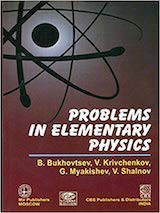# Problems in Elementary Physics by BukhovtsevProblems in Elementary Physics by Bukhovtsev was published by MIR publishers (Russia) in 1971. The book is a collection of 816 problems and their solutions. The book is divided into six chapters

1. Mechanics (290 problems)
2. Heat and molecular physics (104 problems)
3. Electricity and magnetism (226 problems)
4. Oscillations and waves (40 problems)
5. Geometrical optics (102 problems)
6. Physical optics (54 problems)
The content and nature of the problems and their arrangement conform to Textbook on Elementary Physics by GS Landsberg. You can Download Problems in Elementry Physics by Bukhovtsev (archive.org) or buy paperback from Amazon.

## Key Features

The problems in this book require a fundamental knowledge of the basic laws of physics, and the ability to apply them in the most diverse conditions. A number of problems in the book have been revised from those used at the annual contests organized by the Physics faculty of the Moscow University.

The solutions of all the problems are given in great detail. These solutions are a great help to understand all the critical points of a problem.

## Sample Problems

EACH CHAPTER in Bukhovtsev is divided into multiple sections. The problems in each section are arranged in ascending order of complexity. Many problems are conceptual and don't need mathematical skills. Consider this problem from section 1.4 The Law of Conservation of Momentum:

Problem 74: Does a homogeneous disk revolving about its axis have any momentum? The axis of the disk is stationary.

MANY PROBLEMS ARE INTUITIVE and needs lots of imagination and thinking. Consider this problem in section 1.7 Kinematics of Curvilinear Motion (This problem was also asked in IIT JEE):

Problem 160: Four tortoises are at the four corners of a square with a side a. They begin to move at the same time with a constant speed v, the first one always moving in a direction toward the second, the second toward the third, the third toward the fourth and the fourth toward the first. Will the tortoises meet, and if they do, in what time?

THERE ARE PLENTY of problems that requires messy algebraic manipulations. Most of the problem don't need calculus (unlike Irodov) but there is extensive use of algebra, geometry and trigonometry. Consider this problems from section 1.8 Dynamics of Curvilinear Motion:

Problem 218: A double-step pulley consists of two rigidly connected thin hoops with radii R and r and masses M1 and M2 respectively. Strings carrying weights m1 and m2, at their ends are passed around each step (Fig. 92). Find the acceleration of the weights m1 and m2, the tension of the strings and the force with which the system acts on the axis of the pulley.## Summary

The books contains 816 solved problems. Although problems are from elementary physics, but the problems themselves are not elementary. There is good mix of problems that needs conceptual clarity and problem solving skills. Many problems needs command over albebra and geometry but don't need calculus. The book is good but not a first choice for IIT-JEE. Given a choice between Irodov and Bukhovtsev, I will go with Irodov.